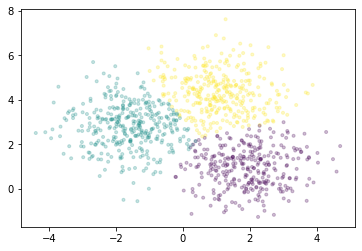# Train Models on Large Datasets¶

Most estimators in scikit-learn are designed to work with NumPy arrays or scipy sparse matricies. These data structures must fit in the RAM on a single machine.

Estimators implemented in Dask-ML work well with Dask Arrays and DataFrames. This can be much larger than a single machine’s RAM. They can be distributed in memory on a cluster of machines.

:

%matplotlib inline

:

from dask.distributed import Client

# Scale up: connect to your own cluster with more resources
n_workers=1, memory_limit='2GB')
client

:


### Cluster

• Workers: 1
• Cores: 4
• Memory: 2.00 GB
:

import dask_ml.datasets
import matplotlib.pyplot as plt


In this example, we’ll use dask_ml.datasets.make_blobs to generate some random dask arrays.

:

# Scale up: increase n_samples or n_features
chunks=100000,
random_state=0,
centers=3)
X = X.persist()
X

:

 Array Chunk 16.00 MB 1.60 MB (1000000, 2) (100000, 2) 10 Tasks 10 Chunks float64 numpy.ndarray

We’ll use the k-means implemented in Dask-ML to cluster the points. It uses the k-means|| (read: “k-means parallel”) initialization algorithm, which scales better than k-means++. All of the computation, both during and after initialization, can be done in parallel.

:

km = dask_ml.cluster.KMeans(n_clusters=3, init_max_iter=2, oversampling_factor=10)
km.fit(X)

:

KMeans(init_max_iter=2, n_clusters=3, oversampling_factor=10)


We’ll plot a sample of points, colored by the cluster each falls into.

:

fig, ax = plt.subplots()
ax.scatter(X[::1000, 0], X[::1000, 1], marker='.', c=km.labels_[::1000],
cmap='viridis', alpha=0.25);For all the estimators implemented in Dask-ML, see the API documentation.